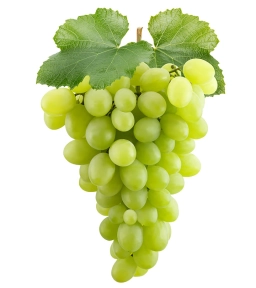# Fruits

2/3 of the fruits are cherries, and the rest are grapes. I sold 1/2 of the cherries and 1/4 of the grapes. If I had 280 left, how many fruits had I at first?

x =  480

### Step-by-step explanation:Did you find an error or inaccuracy? Feel free to write us. Thank you!

Tips for related online calculators
Need help calculating sum, simplifying, or multiplying fractions? Try our fraction calculator.
Do you have a linear equation or system of equations and looking for its solution? Or do you have a quadratic equation?